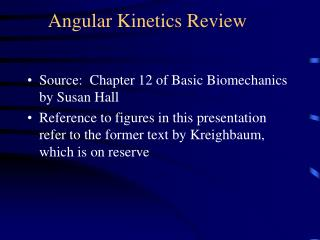DownloadDownload PresentationAngular Kinetics Review

# Angular Kinetics Review

Download Presentation## Angular Kinetics Review

- - - - - - - - - - - - - - - - - - - - - - - - - - - E N D - - - - - - - - - - - - - - - - - - - - - - - - - - -
##### Presentation Transcript

1. Angular Kinetics Review • Source: Chapter 12 of Basic Biomechanics by Susan Hall • Reference to figures in this presentation refer to the former text by Kreighbaum, which is on reserve

2. Torque and Motion Relationships • Relationship between linear and angular motion • displacement, velocity, and acceleration (Fig H.1, p 315) • Angular analogue of Newton’s third law (F=ma), the instantaneous effect of a force or torque • Torque = moment of inertia (I) X angular acc ( (Fig H.5-H.7) • What is torque? • What is moment of inertia ?(Fig H.3, p 319) • What is radius of gyration (Fig H.4, p 320) • Changing moment of inertia and radius of gyration in the body (Figures H.8 and H.9, p 323 and 324) • Calculations using a 3-segment system • Homework problem

3. Instnataneous effect of net torque: Moment of Inertia Constant T = I What is torque?

4. Instantaneous effect of net torque: Torque is constant What is rotational inertia, Or moment of inertia?

5. Instantaneous effect of net torque: Ang acc constant

6. What is Moment of Inertia? It is the resistance of a system to rotational acceleration, and is calculated at follows: Here, r (the radius of rotation) is equal to k (the radius of gyration), but that is not the case with extended bodies

7. What is radius of gyration (k)? k 35 An indicator of distribution of mass about the axis. It is the distance from the axis to a point at which all the mass of a system of equal mass would be concentrated to have the MOI equal the original system. It is, then, the average weighted distance of the mass of a system to the axis. Equivalent systems k 35

8. Determining MOI & K • Simple 3-segment system: • I = 3mi di2 = m1 d12 + m2 d22+ m3 d32 + . . . . . . .+ mi di2 • I = mk2 ; k = (I/m).5 • Irregularly shaped bodies But we can’t measure all of these small masses!

9. Physical pendulum method of determining MOI and K • Suspend object at axis • Measure mass (m), and distance from axis to COM, r • Measure period of oscillation (T) • Moment of inertia (I) = T2 mr * .248387 m/sec • Radius of gyration (K) = ( I/m).5

10. MOI & K – Geometric Objects

11. ChangingIandk in the human body

12. ChangingIand k in the human body

13. Angular Momentum • Impulse-momentum relationship - effect of force or torque applied over time • Linear: Ft = mv Rotational: Tt = I  • What is angular impulse? (Fig I.1, I.2, I.3, p 327-8) • Torque X time • What is angular momentum? (Fig I.4, p 329) • amount of angular movement: I  • Conservation of angular momentum (Fig I.4, I.5, I.6 p 329-331) • Angular momentum is constant if net impulse is zero

14. What is angular impulse?

15. Angular Impulse: Mediolateral axis

16. Angular Impulse around vertical axis

17. What is angular momentum (L)?

18. Conservation of Momentum

19. Conservation of Momentum